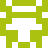### Real Vim ninjas count every keystroke - do you?

###### Pick a challenge, fire up Vim, and show us what you got.

```Your VimGolf key: please sign in

\$ gem install vimgolf
\$ vimgolf setup
\$ vimgolf put 9v0064a68333000000000254
```

### Find fix point of cos

Write a function to find the fix point of cos. It is not a good code; It is just to use vim. Inspired by the excellent https://mvanier.livejournal.com/2897.html about the Y combinator.

##### Start file
```from math import cos

def find_cos_fix_point(j):
a = 12.216
for i in range(j):
a = cos(a)

print(a)

find_cos_fix_point(1)
```
##### End file
```from math import cos

def find_cos_fix_point(j):
a = 12.216
for i in range(j):
a = cos(a)

print(a)

find_cos_fix_point(1)
find_cos_fix_point(10)
find_cos_fix_point(100)
find_cos_fix_point(1000)
find_cos_fix_point(10000)
find_cos_fix_point(100000)
find_cos_fix_point(1000000)
find_cos_fix_point(10000000)
find_cos_fix_point(100000000)
```

#### View Diff

```12a13,20
> find_cos_fix_point(10)
> find_cos_fix_point(100)
> find_cos_fix_point(1000)
> find_cos_fix_point(10000)
> find_cos_fix_point(100000)
> find_cos_fix_point(1000000)
> find_cos_fix_point(10000000)
> find_cos_fix_point(100000000)
```

### Solutions by @r4d1um:

Unlock 8 remaining solutions by signing in and submitting your own entry

## 185 active golfers, 661 entries

##### Solutions by @r4d1um:14
###### #14 - r4d1um / @r4d1um

08/10/2023 at 08:16PM15
###### #>77 - r4d1um / @r4d1um

08/10/2023 at 04:46PM16
###### #>115 - r4d1um / @r4d1um

08/10/2023 at 04:44PM17
###### #>122 - r4d1um / @r4d1um

08/10/2023 at 04:36PM18
###### #>127 - r4d1um / @r4d1um

08/10/2023 at 04:34PM19
###### #>129 - r4d1um / @r4d1um

08/10/2023 at 04:32PM22
###### #>138 - r4d1um / @r4d1um

08/10/2023 at 04:25PM42
###### #>159 - r4d1um / @r4d1um

08/10/2023 at 04:24PM